Next: The protocol Up: CSP Previous: CSP

## Traces

In [Sch96], the traces model is used as the basis for the proof rules presented. In this model, the semantics of a process P is defined to be the set of traces (sequences of events) that it may possibly perform. For example,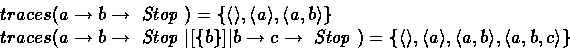A useful operator on traces is projection: If D is a set of events then the trace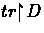is defined to be the maximal subsequence of tr all of whose events are drawn from D . If D is a singleton set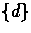then we overload notation and writefor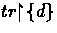. Message extraction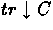for a set of channel names C provides the maximal sequence of messages passed on channels C . Finally,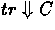provides the set of messages in tr passed along some channel in C . These may be described inductively on sequences, and the last by a set comprehension: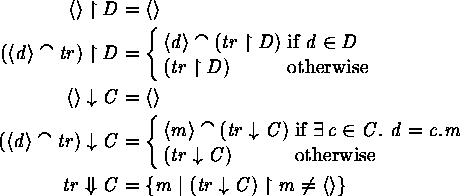If tr is a sequence, then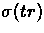is the set of events appearing in the sequence. The operator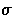extends to processes: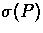is the set of events that appear in some trace of P .

In the traces model, if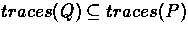then we say that Q is a refinement of P , written.Next: The protocol Up: CSP Previous: CSP